Phased Array
This is a simulation of the behaviour of a linear phased array antena. s an implementation of the formulae given in "An Introduction to Radar Systems " M Skolnik.
This is a linear array of "Nel " elements, seperated by a distance of "d" meters and let the wavelenght of the signal will have be " l " centimeters.
If we want to deflect the beam by an angle "q " relative to the perpedicular, we must feed each element with a signal that is phase shifted by an angle "y " releative to its neighbour.
So:
WavelengthElement seperationNumber of elementsAngle of deflectionPhase shift between elements, y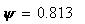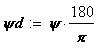The normalised gain in terms of angle from the perpendicular is given by:Also let's compute the Phase shift between elements for linear array of N elements.
Describe viriable parameters: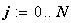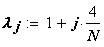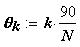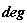Calculate equation: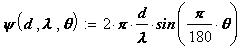Phase shift between elements
Create plots: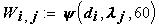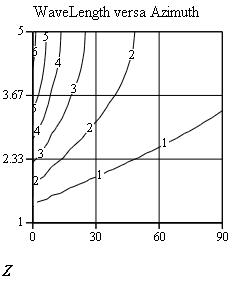We can plot the response for normalised gainas for follows: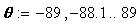Mathcad file developed by Roman N Tunkel , March.15/2002
C3I, Raytheon Systems Company. roman_n_tunkelr@raytheon.com